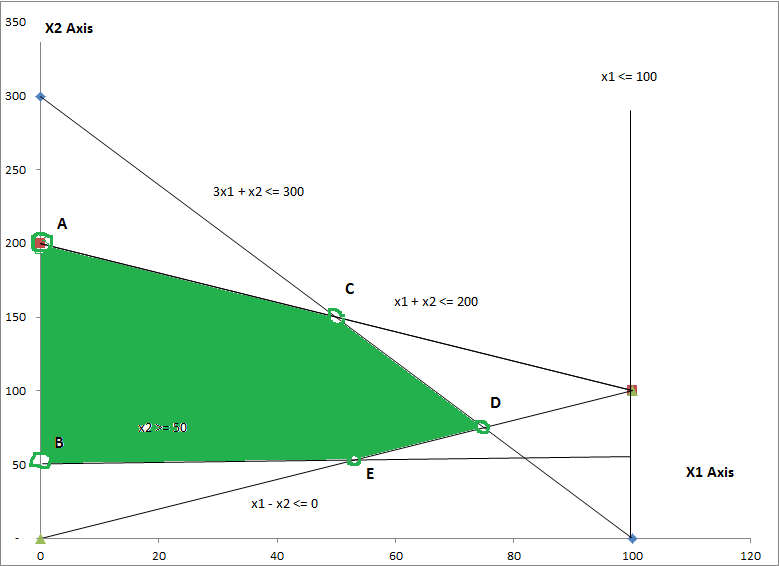# Question & Answer: Maximize Z= 15X1 +15X2 Subject​ to: C1 3×1 + 1×2…..

 Maximize Z= 15X1 +15X2 Subject​ to: C1 3×1 + 1×2 <= 300 C2 1×1 + 1×2 <= 200 Don't use plagiarized sources. Get Your Custom Essay on Question & Answer: Maximize Z= 15X1 +15X2 Subject​ to: C1 3×1 + 1×2….. GET AN ESSAY WRITTEN FOR YOU FROM AS LOW AS \$13/PAGE C3 1×1<=100 C4 1×2>=50 C5 1×1 – 1×2 <= 0 x1, x2 >= 0 The optimum solution: x1: x2: Optimal solution for Z:

Using graphical method:The 5 points and Z value is:

A = 0,200

Z = 15*0 + 15*200 = 3000

B = 0,50

Z = 15*0 + 15*50 = 750

C = Intersection of 3×1 + x2 <= 300 & x1 + x2 <= 200

C = 50,150

Z = 15*50 + 15*150 = 3000

D = Intersection of 3×1 + x2 <= 300 & x1 – x2 <= 0

D = 75,75

Z = 15*75 + 15*75 = 2250

E = 50,50

Z = 15*50 + 15*50 = 1500

Thus, both A & C points are optimum solution